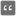• # What's wrong with this code output is "undefined"

## Question related to missionEnd Zeros

def end_zeros(num: int) -> int: x = 0 while True: if num % 10 != 0: return x elif num % 10 == 0: num //= 10 x += 1 continue
return x

if name == "main": print("Example:") print(end_zeros(0))

```# These "asserts" are used for self-checking and not for an auto-testing
assert end_zeros(0) == 1
assert end_zeros(1) == 0
assert end_zeros(10) == 1
assert end_zeros(101) == 0
assert end_zeros(245) == 0
assert end_zeros(100100) == 2
print("Coding complete? Click 'Check' to earn cool rewards!")
```